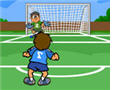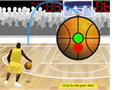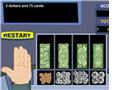# Subtraction Games

Are you looking for free subtraction games that you or your students can play online? On this page we have cool math games that provide lots of opportunity to practice subtraction problems with whole numbers, decimals, fractions, integers, and money.

Take advantage of our free subtraction games and sharpen your math skills today!Subtracting Two-Digit Numbers

In this interactive soccer game, kids will practice adding two-digit whole numbers.Baseball Math - Subtracting Decimals

When playing this fun baseball math game, elementary students can earn points for hitting homeruns and for correctly solving different subtraction problems.Basketball Math - Subtracting Decimals

Students everywhere can sharpen their subtraction skills by playing this fun basketball game alone or with a partner.Soccer Math Game - Subtracting Decimals

How many goals can you score? You must answer each subtraction problem correctly to have a chance to kick the ball and score goals.Math Racing - Subtracting Integers Game

This is an exciting car racing game about subtracting positive and negative integers.Subtracting Money Game

In this interactive game, students will solve simple word problems about subtracting money by using virtual coins and bills.

Return from the Subtraction Games page to the Math Play homepage or to the Elementary Math Games page.